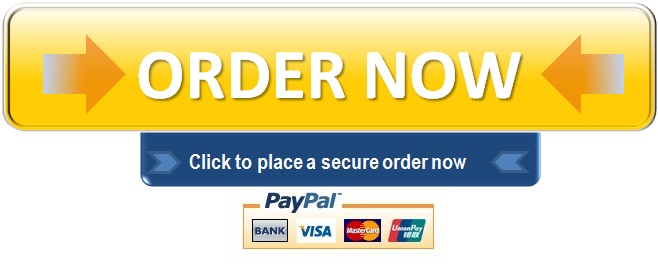The objective of the teacher during the mathematics lesson was to teach the students how to add and subtract. He ensured that the students can identify the keywords and knew whether they are required to add or subtract. For example, the teacher asked the students, “If a person gave away ten pencils, how many is left?” students were left to think and determine if the concept entails addition or subtraction. However, the gradual release of responsibility was evidenced when the teacher gave an independent practice to the students. The students were given an opportunity to apply what they had learned in a new way by playing online games. Therefore, students were asked to play at least three online games to solve mathematics. Also, they were required to get at least 80% as the score for the games played. Additionally, students were placed in small groups so that they could work collaboratively and achieve their target goal. The teacher moved around the class guiding those students who required his help and also he gave out further instructions.

On the other hand, the feedback concept is evidenced in the following way. The online games students played using the computers, and IPads provided immediate feedback and gave the scores that the students had obtained. Also, the teacher kept on moving around to give his feedback to the students. Assessment is much critical to students during their learning periods. Therefore, students were given benchmark exams at the end of the year. It helps in monitoring their learning progress.

Teachers find it Ideal to use technology in their lessons. For this particular case, the teacher had to find a way of incorporating technology into the lessons. The teacher’s objective was to ensure that the students have understood the concept of addition and subtraction before moving to technology. He asked the students to tell how they knew it was an addition math. The students responded by giving the correct answer hence he became sure that the students have understood. The understanding by the students made the teacher move to the use of the computer. Differentiation allows the students to learn at a speed that they find to be comfortable (Heacox, 2012). Therefore, the students will learn the concepts in different ways and speed. Similarly, the teacher will use technology to pace lesson for students according to their degree of learning.

The learners had a varied degree of understanding the mathematics concepts. Some students were not in a position to raise their hands to show that they can answer the questions. Also, the teacher was forced to work with some students in small groups to give them support. The first level of depth of knowledge is recall and production. The students portrayed this level by calculating the basic mathematics of addition and subtraction. The second level is working with skills and concepts. For example, the students explained why the question posed by the teacher was an addition or subtraction concept.

I believe that the students have faith with this kind of learning environment, and they think they can solve the mathematics problems. Students have shown confidence by answering the questions posed by the teacher. The use of technology to teach students has motivated them to love math. Also, the students can interact with one another through discussing in small groups. Similarly, they are always given support from their teacher in case they do not understand the concepts well.

References

Heacox, D. (2012). Differentiating instruction in the regular classroom: How to reach and teach all learners (Updated anniversary edition). Free Spirit Publishing.

Do you need an Original High Quality Academic Custom Essay?